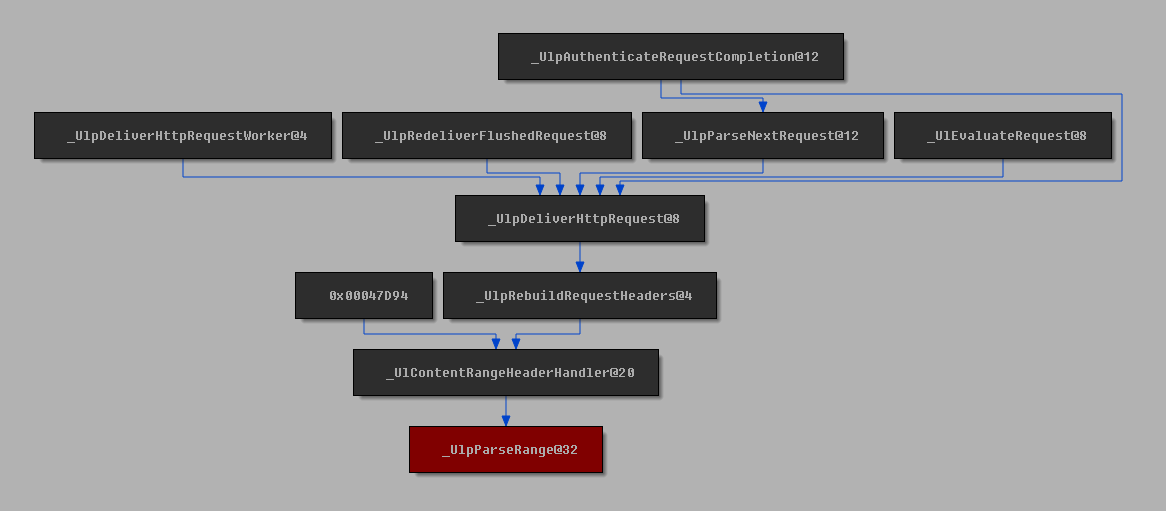# Plotting a Call Graph¶

## Usage¶

Using Windows 8.1 `http.sys`. Before `MS15-034`.

```draw_call_graph(sark.Function(name="_UlpParseRange@32").ea, to=True, distance=4)
```## Code¶

```import sark
import networkx as nx

def draw_call_graph(ea, distance=2, to=False):
# First, get the IDB graph (caching it might be a good idea
# as this operation can be time consuming on large IDBs)
idb_graph = sark.graph.get_idb_graph()

# Get the address of the function to use in the graph
func_ea = sark.Function(ea).ea

# Create the call graph
if to:
# If we want the calls to our function, we need to reverse
# the graph
idb_graph = idb_graph.reverse()

# Use NetworkX to limit the IDB graph
call_graph = nx.ego_graph(idb_graph, func_ea, distance)

# Paint the root node red
call_graph.node[func_ea][sark.ui.NXGraph.BG_COLOR] = 0x80

if to:
# If we reversed it before, we need to reverse it again
# to make the links point the right way
call_graph = call_graph.reverse()

# Create an NXGraph viewer
viewer = sark.ui.NXGraph(call_graph, handler=sark.ui.AddressNodeHandler())

# Show the graph
viewer.Show()
```